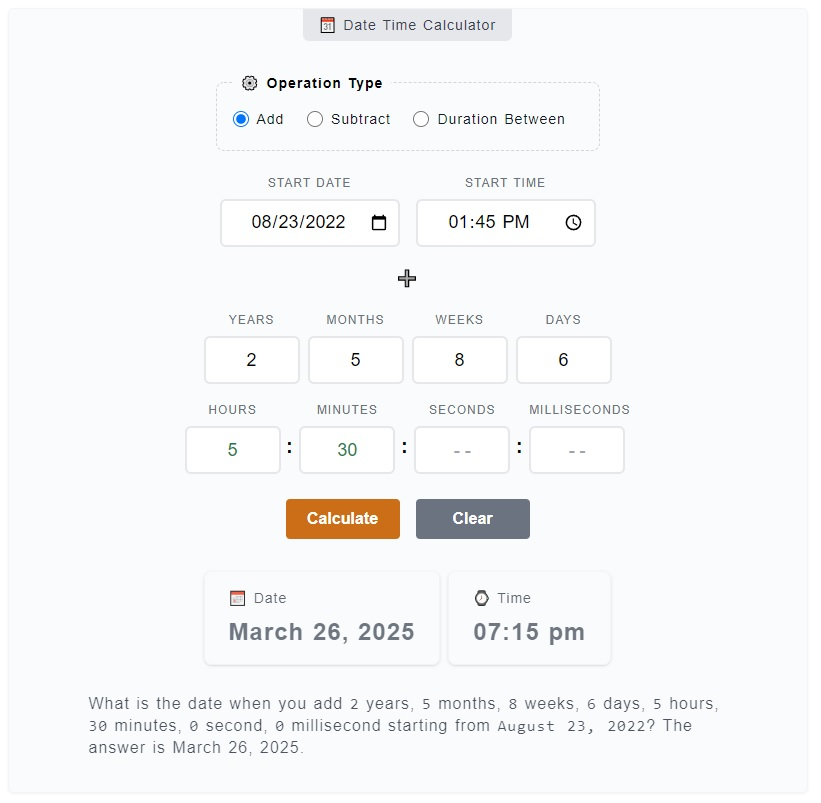# Date Time Calculator

The Date Time Calculator is used to perform basic date and time operations where you can add, subtract and find the duration between two dates and times. You just have to pick the right operation type and enter the date and time. There are also separate calculators built for in depth functionalities - Date Calculator and Time Calculator.

🗓️ Date Time Calculator
⚙️ Operation Type
:
:
:

June 23, 2023

### ⌚ Time

07:04 am

What is the date when you add `0` year, `0` month, `0` week, `0` day, `0` hour, `0` minute, `0` second, `0` millisecond starting from `June 23, 2023`? The answer is .

## How to Use the Date Time Calculator

There are instances that you really need help from counting-off days, or finding what's the entire duration between two date and times. These things are much better solved using this `Date Time Calculator` than doing them manually.

For example, you want to know how many days are there starting from January 1, 2023 with the current local time and December 24, 2024 at exactly 12:00 midnight. With this calculator, you'll have the exact details you are looking for.

The calculator is grouped into three (3) parts, the first set is marked with `⚙️ Operation Type` where you can select the operation you want to perform (functionality). The second set is the main one where there's input fields and buttons. The third is the result area where it displays the outcome of the calculations.

### Add Date and Time Usage

Here's a step by step guide on how to use the Data and Time Calculator in order to `Add Date and Time`:1. Step 1

On the topmost part of the calculator, you can find the `⚙️ Operation Type` field set. It is where you need to choose from the operation options - add, subtract and duration between. If you want to add days, weeks, months and/or years, select the `add` radio button.

2. Step 2

After you have chosen the `Add` operation (which is selected by default), you'll get to enter the `Start Date` and `Start Time`. This date and time servers as a reference when we add the days, months, etc. Let's make an example, enter as start date and as start time.

3. Step 3

When you have entered the `Start Date` and `Start Time`, you can now enter the days, months, weeks, days, hours, minutes, seconds and milliseconds that you want to be added to the start date and time. You can choose the leave inputs blank if you don't need them. Continuing our example on step 2, let's add `4 months`, `6 days` and `10 hours`.

4. Step 4

The final step for adding date and time is to click the `Calculate` button. The results will be displayed below the buttons. You can click the `Clear` button to reset the input fields. Following our example so far, the answer should be at .

### Subtract Date and Time Usage

Here's a step by step guide on how to use the Data and Time Calculator in order to `Subtract Date and Time`:

1. Step 1

On the `⚙️ Operation Type` field set, click on the `Subtract` radio button for we want to subtract days, months and time from a start date and time.

2. Step 2

The change to `subtract` operation type is confirmed by the visibility of subtract (➖) sign. What you need to do now is to enter the `Start Date` and/or `Start Time`. It's best to give an example inputs here, let's enter as start date and as start time.

3. Step 3

After you have entered the `Start Date and Time`, you can now enter the days, weeks, months, years, hours, minutes, seconds and milliseconds that you want to subtract from the reference date and time. For the fields that are not needed, you can just leave them blank. Continuing our example on step 2, let's subtract `7 years`, `3 weeks`, `5 days` and `6 hours`.

4. Step 4

The final step for adding date and time is to click the `Calculate` button. The results will be displayed below the buttons. You can click the `Clear` button to reset the input fields. Following our example, the answer should be at .

### Duration Between Date and Time Usage

Here's a step by step guide on how to use the Data and Time Calculator in order to `Duration Between Date and Time`:

1. Step 1

On the `⚙️ Operation Type` field set, click on the `Duration Between` radio button for we want to get the entire duration between from the start date and time to the end date and time.

2. Step 2

The `duration between` operation type is confirmed by arrow sign (⇢). What you need to do now is to enter the `Start Date` and/or `Start Time`. Let's try entering as start date and as start time.

3. Step 3

Then, enter the `End Date` and `End Time` on their corresponding date and time input fields. They are labeled so that it will be easier for you to distinguish them. Continuing with our example, let's enter as end date and as end time.

4. Step 4

The final step for adding date and time is to click the `Calculate` button. The results will be displayed below the buttons. You can click the `Clear` button to reset the input fields. For our previous example inputs, the answer should be `9 months, 3 weeks and 2 days`. All other totaled minutes, hours, days, etc. should also be displayed.

## Video Explanation

Here's a video tutorial on how to use the Date Time Calculator.